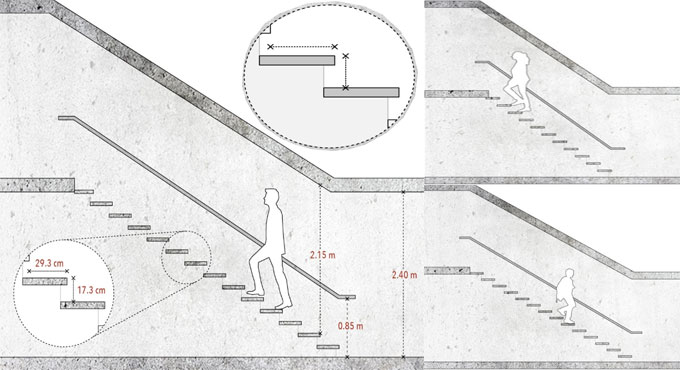# Some vital guidelines for measuring staircase dimensions and designsA staircase mainly includes a series of steps which involve a tread (the horizontal portion, where the foot stands) and a riser (the vertical portion).

In every step, there are one or more landings, handrails, and a small nosing. The latter obtrudes from the tread over the lower step, facilitating to raise its size devoid of inclusion of centimeters to the overall dimensions of the staircase.

By using the following formula, find out the exact dimensions of a convenient and efficient staircase in accordance with its use.

2 Risers + 1 Tread = 63-65 cm

The required space to attain these optimal dimensions is unavailable sometimes, but it's suggested to approach them as much as possible.

A schematic illustration of a steep and low-transit staircase.

(2 x 21) + (1 x 21) = 63 cm

A schematic illustration of an optimal staircase.
(2 x 18) + (1 x 28) = 64 cm

A schematic illustration of a loose staircase, desirably for exterior application.
(2 x 13) + (1 x 39) = 65 cm

Sample measurement of a staircase that should be 2.60 meters high.

1. Workout the required number of steps - Assume an ideal riser of 18 cm, the height of the space is divided with the height of each step. The result should always be rounded up:
260/18 = 14.44 = 15 steps

2. Workout the height of every riser - The height of the space is divided with the number of steps already acquired:
260/15 = 17.33 cm height for each riser.

3. Workout the width of the tread - It can be calculated with the following formula:
(2 x 17.33 cm) + (1 x tread) = 64

Each tread will be computed as 29.34 cm

The consequential staircase will contain 15 steps of 29.34 cm of tread and 17.33 cm of riser.

Based on the use and local regulations, there should be a minimum width of 80 cm for stairs in single-family homes, and more than 1.00 meters in public buildings.

Preferably, a stairway shouldn't contain in excess of 15 steps in a row. After 15 steps, a landing should be arranged. It's suggested that a landing is calculated minimum the same as 3 treads.

The height among the steps and the ceiling should remain 2.15 meters at minimum. The height of the handrail differs among 80 and 90 cm from each step.